# NCERT Solutions for Class 10 Maths Exercise 7.3

Get Free NCERT Solutions for Class 10 Maths Chapter 7 Ex 7.3 Coordinate Geometry Class 10 Maths NCERT Solutions are extremely helpful while doing homework. Exercise 7.3 Class 10 Maths NCERT Solutions were prepared by Experienced ncertlive.in Teachers. Detailed answers of all the questions in Chapter 7 Maths Class 10 Coordinate Geometry Exercise 7.3 Provided in NCERT Textbook

NCERT Solutions For Class 10 Maths Chapter 7 Coordinate Geometry Ex 7.3
NCERT Solutions for Class 10 Maths Chapter 7 Coordinate Geometry Ex 7.3 are part of NCERT Solutions for Class 10 Maths. Here we have given NCERT Solutions for Class 10 Maths Chapter 7 Coordinate Geometry Ex 7.3.

## Exercise 7.3 Class 10 NCERT Solutions

Ex 7.3 Class 10 Maths Question 1.
Find the area of the triangle whose vertices are: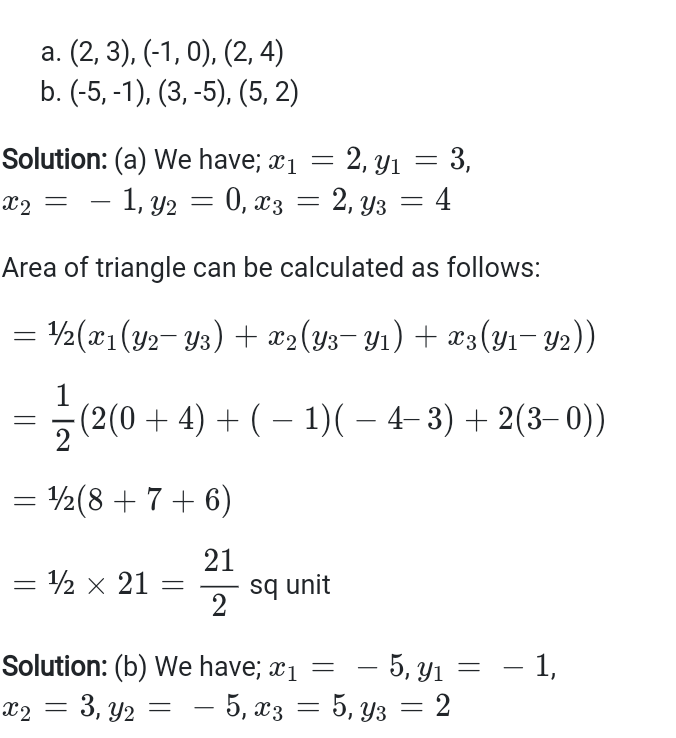Ex 7.3 Class 10 Maths Question 2.
In each of the following find the value of ‘k’, for which the points are collinear.
(i) (7, -2), (5, 1), (3, -k)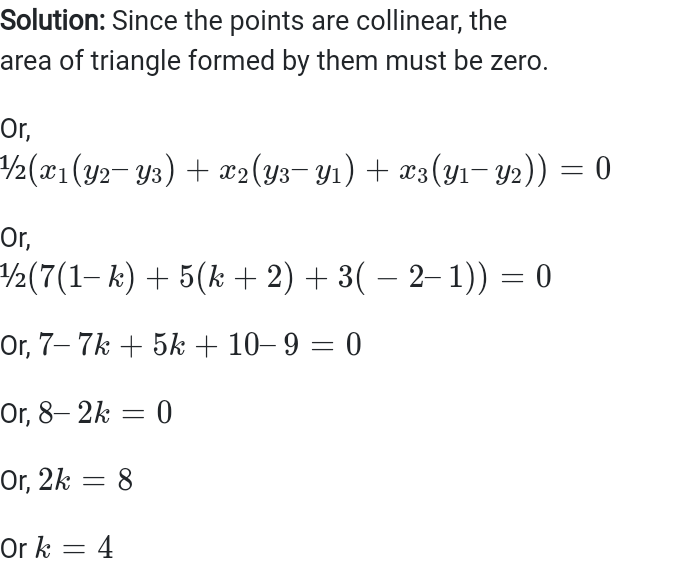(ii) (8, 1), (k, -4), (2, -5)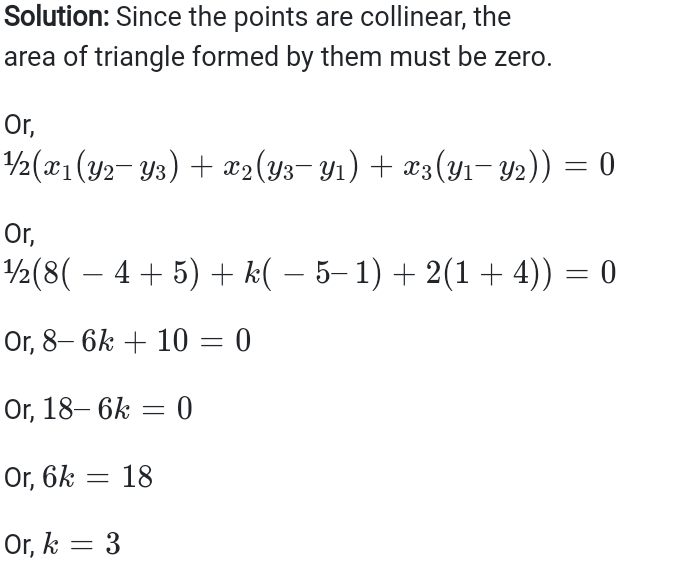Ex 7.3 Class 10 Maths Question 3.
Find the area of the triangle formed by joining the mid-points of the sides of the triangle whose vertices are (0, -1), (2, 1) and (0, 3). Find the ratio of this area to the area of the given triangle.
Ex 7.3 Class 10 Maths Question 4.
Find the area of the quadrilateral whose vertices, taken in order, are
(-4, -2), (-3, -5), (3, -2) and (2, 3).
Ex 7.3 Class 10 Maths Question 5.
You have studied in Class IX that a median of a triangle divides it into two triangles of equal areas. Verify this result for ΔABC whose vertices are A (4, – 6), B (3, – 2) and C (5, 2).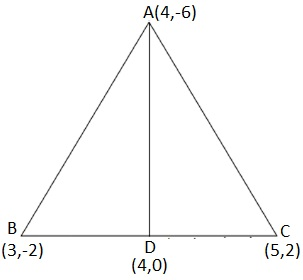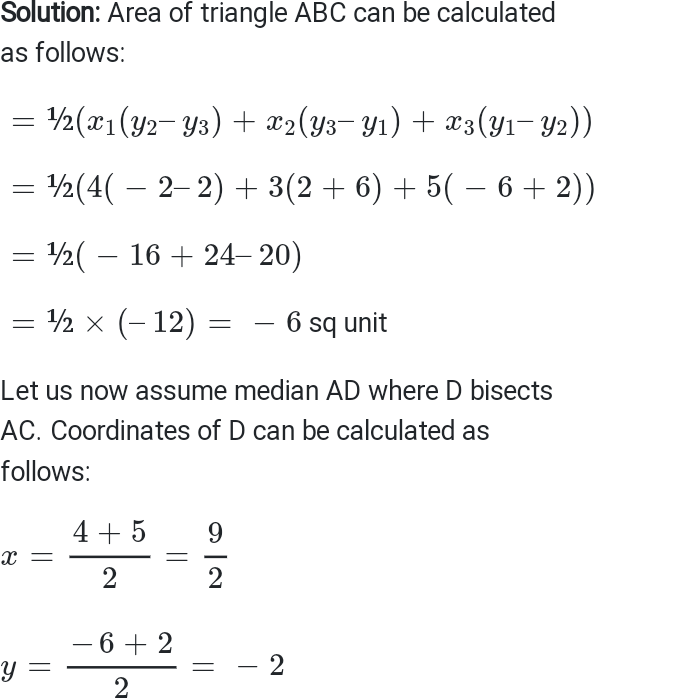Access Other Exercise Solutions of Class 10 Maths Chapter 7- Coordinate Geometry
Exercise 7.1– 10 Questions (8 practical based questions, 2 reasoning questions)
Exercise 7.2– 10 Questions (8 long answer questions, 2 short answer questions)
Exercise 7.4– 8 Questions (6 long answer questions, 1 practical based question, 1 reasoning question)

NCERT exercise Solutions provided here ensures that you get all the vital information on calculating Areas of a triangle. Areas of a triangle are very important from the point of CBSE Class 10 first term examination. Hence, students are advised to get familiar with the formulas and methods of calculating areas of triangles.

A great way of learning Maths is by solving the problems. To make you learn the methods of calculating areas of triangle, we have provided detailed solutions to questions provided in Exercise 7.3 of NCERT class 10 textbook.

Topics covered in Exercise 7.3
1. Section formula
2. Solved examples